# Tricks to Solve Profit and Loss Questions: Part-2

• Cost Price: The price, at which an article is purchased, is called its cost price or C.P.
• Selling Price: The price, at which an article is sold, is called its selling price or S.P.
• Profit or Gain: If S.P. is greater than C.P., the seller is said to have a profit or gain. S.P.>C.P.
• Loss: If S.P. is less than C.P., the seller is said to have incurred a loss. C.P.>S.P.

### 1.

Profit= Selling Price - Cost Price
Example:  If the S.P. of a shirt is 500 Rs. and C.P. is 425 Rs. Find the Profit or Loss.
Solution: S.P.>C.P. (Profit)
Profit= Selling Price - Cost Price
Profit= 500-425
Profit=75 Rs.

### 2.

Loss= Cost Price - Selling Price
Example: If the shopkeeper purchased a dozen copy for 360 Rs. and sold 7 copies for 175 Rs. What is the total Profit/Loss of 8 copies?
Solution: C.P. of a copy= 360/12 = 30 Rs.
S.P. of a copy= 175/7 = 25 Rs.
C.P>S.P. (Loss)
Loss= Cost Price - Selling Price
Loss= 30-25
Loss = 5 Rs.
5×8 = 40 Rs.

### 3.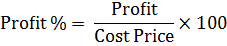Example: If the S.P. of a shirt is 625 Rs and C.P. is 500 Rs. What is the Profit %?
Profit = 625-500 =125 Rs.Profit % = 25%

### 4.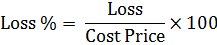Example: If a person purchased a cycle for 2000 Rs. and sold it at 15% loss. Find the Loss.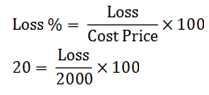Loss= 400 Rs.

### 5.Example:  If Mohan purchased a table for 400 Rs. and sold it to Sohan at 10% profit. What is the S.P.?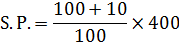S.P. = 440 Rs.

### 6.Example:  If Aabha purchased a cooler for 600 Rs. and sold it to Sohan at 15% loss. What is the S.P.?S.P. = 510 Rs.

### 7.Example:  Navya sold a computer for 22000 Rs. at 10% profit. What is the C.P of that computer?
Solution:Example:  Saumya sold his plot for 2,00,000 Rs. at 20% loss. Find the C.P.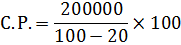C.P. = 250000 Rs.

### 9.Example:  M.R.P of an article is 20% more than the C.P. If the C.P. of that article is 200 Rs. and a person purchased it after discount of 36 Rs. Find the Discount %.
Solution:C.P. = 200, M.R.P. = 200×120%=240
=15%

### 10.Example:  M.R.P of an article is 15% more than the C.P. If the C.P. of that article is 300 Rs. and a shopkeeper sold it after 10% discount. What is the S.P. of that article?
Solution: C.P. =300, M.R.P. = 300×115% = 345S.P. = 345×90/100
S.P. =310.5 Rs.